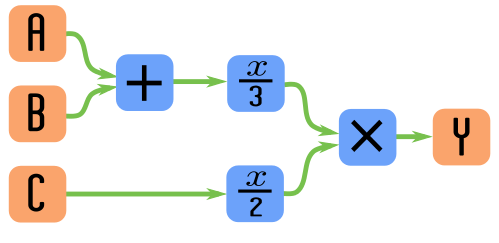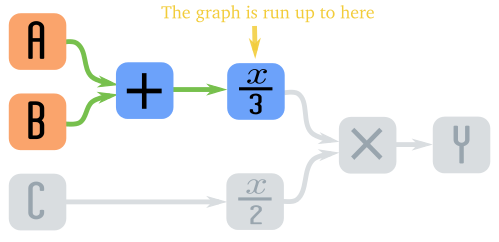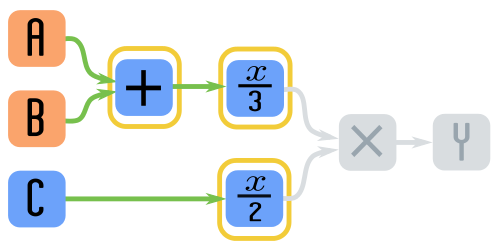# 01 Graphs and Sessions

In the intro page, you were introduced to the following template of code.

```from __future__ import print_function, division
import tensorflow as tf
import numpy as np

# ------------------------------------------------
#                                    Build a graph
# ------------------------------------------------
graph = tf.Graph()
with graph.as_default():
pass # REPLACE WITH YOUR CODE TO BUILD A GRAPH

# ------------------------------------------------
#              Create a session and run the graph
# ------------------------------------------------
with tf.Session(graph=graph) as session:
pass # REPLACE WITH YOUR CODE TO RUN A SESSION
```

Apart from the imports of libraries, you will notice that the template consists of two components:

• Building a graph
• Running a session

These will be explained in more detail in the following sections of this lesson.

## Graph

In order to make use of Tensorflow, we must first create what is called a graph. A graph is a set of operations that are connected together in some way. For example, the diagram below shows the graph representation of this formula `y=(A+B)3*(C/2)`We see the inputs A, B, and C. Their values travel along the green lines (called the edges of the graph). And on the blue nodes, we apply some operation to those values coming in.

In the context of Neural Networks, this can be thought of as creating the architecture of our neural network. So here we specify the inputs, the layers, nonlinearities, loss functions, and optimization operations.

The values that travel along the edges of the graph are tensors (hence the name Tensorflow), which can be thought of as multi-dimensional arrays, such that:

• 0 Dimensional Tensor: is equivalent to a scalar value (eg a float or an integer)
• 1 Dimensional Tensor: is equivalent to a vector.
• 2 Dimensional Tensor: is equivalent to a matrix.
• N-Dimensional Tensor: extends the concept of a matrix to N dimensions.

Specifying the graph that is shown in the diagram above can be done as follows. Run the code in the following cell to create the graph.

```graph = tf.Graph()
with graph.as_default():
tf_A = tf.constant(3.0)
tf_B = tf.constant(9.0)
tf_C = tf.constant(4.0)

tf_summed = tf_A + tf_B
tf_div3 = tf.div(tf_summed, 3.0)
tf_div2 = tf.div(tf_C, 2.0)
yf_y = tf.mul(tf_div3, tf_div2)
```

Now, let's try to get the value of the final operation in the graph by running the following code.

```print(tf_y)
```

You might have expected it to output a value of 8.0, but instead, you get a Tensor object.

The reason is that when you specify a tensorflow graph, tensorflow does not actually run the operations, it simply creates a specification for how data should flow. `tf_y` is simply pointing to an operation in the graph, and does not store the output of that operation. In order to actually make the data flow through the graph, we need to initialize and run a session. This will be explained in the following section.

## Session

In order to actually run data through a Tensorflow graph, we need to initialize a session and specify what portion of the graph we want to run. If we wish to run all the operations up to `tf_y` and get the output of `tf_y`, we run:

```with tf.Session(graph=graph) as session:
y = session.run(tf_y)
print(y)
```
```[OUTPUT]
8.0
```

When calling sess.run() we pass it the operation that we are interested in running from the graph. Tensorflow automatically runs any other operations which your desired operation depends on (and only those).

So, for instance, if you specified that you want to run the `tf_div3` operation, then it will also automatically run the `tf_summed` operation, but it will not compute the `tf_div2` or `tf_mult` operations.

```with tf.Session(graph=graph) as sess:
div3 = sess.run(tf_div3)
print(div3)
```
```[OUTPUT]
12.0
```### Returned Values from sess.run()

The first argument that we pass on to sess.run() is the operation we want to run in the graph. The value returned by sess.run() as a numpy object. If the operation evaluates to a scalar value, then sess.run() will return a numpy scalar (like a numpy float).

Run the following code to see the data type of the value of the previous value.

```type(tf_div3)
```
```[OUTPUT]
numpy.float32
```

If the operation in the graph evaluates to a 1D, 2D, or ND tensor, then sess.run() will return a numpy array object.

### Evaluating/Returning Multiple Operations

If you wish to evaluate several operations in the graph or wish to find the values of several parts of the graph, then you can pass a list of operations as the first argument to `sess.run()`.

For example, running the following code will evaluate the `tf_summed`, `tf_div3` and `tf_div2` operations, and return their evaluated values.

```with tf.Session(graph=graph) as sess:
summed, div3, div2 = sess.run([tf_summed, tf_div3, tf_div2])
print(summed)
print(div3)
print(div2)
```
```[OUTPUT]
12.0
4.0
2.0
```## EXTRA: Why Tensorflow splits the process into graph and Session

Python is a wonderful programming language. It is simple and fun to use. However, it is not very fast. Performing math operations on large matrices can be time-consuming.

Many Python libraries for scientific computing have been designed to overcome this issue by being written in some other programming language like C or C++. By performing the calculation in a faster programming language, they speed things up.

You interact with those libraries using python functions as you normally would. But under the hood, it takes the data and performs the operation outside of python, then returns the data back into Python.

This greatly speeds things up. However, there is still some overhead involved in transferring the data in and out of Python. The overhead involved in transferring data is even more pronounced when we are making calculations on a distributed system that spread the computations across multiple computers.

Instead of transferring data in and out of Python after every single operation, Tensorflow will run a series of operations outside of Python. Only after all those operations have been performed will it return back to Python.

This is the point of creating a graph. A graph is a way to specify the series of steps that will need to be calculated. Opening a session creates a connection to the system that will actually perform the calculations. And running a portion of the graph specifies how much of the operations will need to be calculated outside of Python before it returns again.